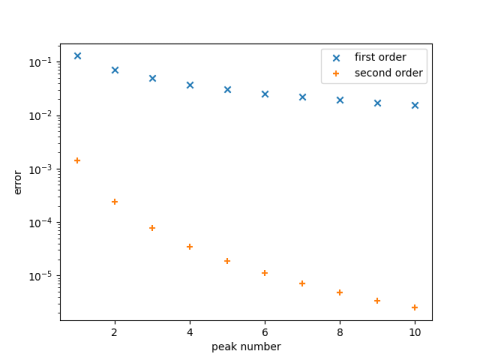# Peaks of Sinc

Yesterday I wrote two posts about finding the peaks of the sinc function. Both focused on numerical methods, the first using a contraction mapping and the second using Newton’s method. This post will focus on the locations of the peaks rather than ways of computing them.

The first post included this discussion of the peak locations.

The peaks of sin(x)/x are approximately at the same positions as sin(x), and so we use (2n + 1/2)π as our initial guess. In fact, all our peaks will be a little to the left of the corresponding peak in the sine function because dividing by x pulls the peak to the left. The larger x is, the less it pulls the root over.

This post will refine the observation above. The paragraph above suggests that for large n, the nth peak is located at approximately (2n + 1/2)π. This is a zeroth order asymptotic approximation. Here we will give a first order asymptotic approximation.

For a fixed positive n, let

θ = (2n + 1/2)π

and let

x = θ + ε

be the location of the nth peak. We will improve or approximation of the location of x by estimating ε.

As described in the first post in this series, setting the derivative of the sinc function to zero says x satisfies

x cos x – sin x = 0.

Therefore

(θ + ε) cos(θ + ε) = sin(θ + ε)

Applying the sum-angle identities for sine and cosine shows

(θ + ε) (cos θ cos ε – sin θ sin ε) = sin θ cos ε + cos θ sin ε

Now sin θ = 1 and cos θ = 0, so

-(θ + ε) sin ε = cos ε.

or

tan ε = -1/(θ + ε).

So far our calculations are exact. You could, for example, solve the equation above for ε using a numerical method. But now we’re going to make a couple very simple approximations . On the left, we will approximate tan ε with ε. On the right, we will approximate ε with 0. This gives us

ε ≈ -1/θ = -1/(2n + 1/2)π.

This says the nth peak is located at approximately

θ – 1/θ

where θ = (2n + 1/2)π. This refines the earlier statement “our peaks will be a little to the left of the corresponding peak in the sine function.” As n gets larger, the term we subtract off gets smaller. This makes the statement above “The larger x is, the less it pulls the root over” more precise.

Now let’s see visually how well our approximation works. The graph below plots the error in approximating the nth peak location by θ and by θ – 1/θ.Note the log scale. The error in approximating the location of the 10th peak by 20.5π is between 0.1 and 0.01, and the error in approximating the location by 20.5π – 1/20.5π is approximately 0.000001.

 As pointed out in the comments, you could a slightly better approximation by not being so simple. Instead of approximating 1/(θ + ε) by 1/θ you could use 1/θ – ε/θ² and solve for ε.

## 3 thoughts on “Peaks of Sinc”

1. I enjoyed reading your blogs. Similar blogs in these subjects are either too simplistic or too complex (either left of the peak or right of the peak:) Your articles are right at the peak of the knowledge sharing. Keep it up.

2. Thanks! That’s what I aim for.

3. If you expand the left-hand side of $\tan \epsilon = -1/(\theta + \epsilon$ to linear-in-epsilon, why not also expand the right hand side to linear-in-epsilon? You get $\epsilon = – \theta / (\theta^2+1)$, which does slightly better than $\epsilon = -1/\theta$.